Tournament

(diff) ← Older revision | Latest revision (diff) | Newer revision → (diff)

An oriented graph (cf. also Graph, oriented) without loops, each pair of vertices of which are joined by an arc in exactly one direction. A tournament withvertices can be regarded as the outcome of a competition withplayers, the rules of which forbid draws. The notion of a tournament is used for orderingobjects by the method of pairwise comparison. In this connection it finds application in biology, sociology, etc.

A tournament is said to be transitive if its vertices can be indexed by the numbersin such a way that there is an arc going from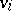to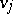if and only if. There are no circuits in a transitive tournament. A tournament is said to be strong if for any ordered pair of vertices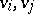there exists a directed path fromto. A set of arcs in a tournament is called compatible if there are no circuits in the subgraph formed by these arcs and the vertices incident to them. The maximum cardinality of a set of compatible arcs is a measure of compatibility in the definition of "the winner" of the tournament. Every tournament contains a subset of compatible arcs of cardinality not less than. Another measure of compatibility is the ratio of the number of transitive-vertex subtournaments of a tournament withvertices to the number of strong-vertex subtournaments. The maximum number of strong-vertex subtournaments of a tournament withvertices is equal to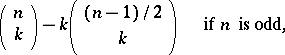A tournament is strong if and only if it has a spanning cycle (Hamiltonian circuit). Every strong tournament withvertices has a circuit of lengthfor. Every tournament has a spanning path (Hamiltonian path).

The outdegreesof a tournament withvertices satisfy the equationSuppose that a set of integerssatisfies the condition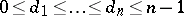. Then a tournament with outdegrees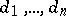exists if and only if for anythe inequality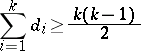holds, with equality for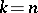. Furthermore, a tournament is strong if and only if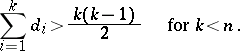Ifand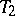are two subtournaments of a tournamentand if there exists an arc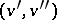for each pair of verticesin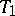andin, then one writes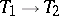. Suppose that the set of vertices of a tournament is partitioned into non-intersecting subsets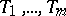. Suppose further that either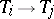or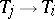for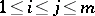. Then the partition defines an equivalence relation on the vertices of. A tournament is called simple if no non-trivial equivalence relation can be defined on its vertices. Every tournament withvertices is a subtournament of some simple tournament with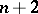vertices. A tournamentwithvertices is a subtournament of some simple tournament withvertices if and only ifis neither a circuit with three vertices nor a non-trivial transitive tournament with an odd number of vertices. The number of pairwise non-isomorphic tournaments withvertices is asymptotically equal to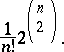The number of distinct tournaments withindexed vertices is equal to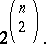The generating functionsand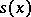for tournaments and strong tournaments, respectively, are related by the formula:Every tournament withvertices,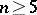, that is not strong is uniquely recoverable from the family of its-vertex subtournaments.

How to Cite This Entry:
Tournament. Encyclopedia of Mathematics. URL: http://encyclopediaofmath.org/index.php?title=Tournament&oldid=11816
This article was adapted from an original article by A.A. Sapozhenko (originator), which appeared in Encyclopedia of Mathematics - ISBN 1402006098. See original article